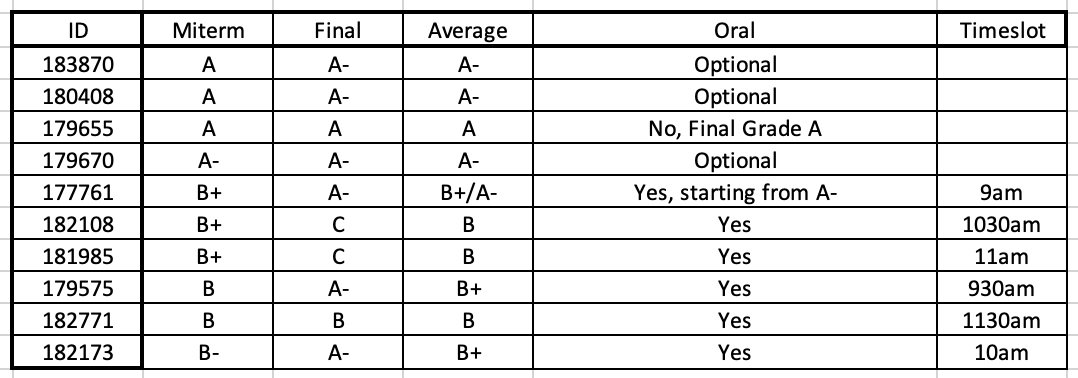Select Page

# ECE231 Introduction to OpticsPlease click here to download an updated sillaby with detailed information on the course.

## Class Notes

 Last Update Topic Summary Slides 4-Sep-2019 Revision of elementary EM Maxwell’s equations in isotropic media. Poynting theorem. Definition and physical meaning of the Poynting vector and the energy density of the electromagnetic wave. Complex formalism. Time average of products of sinusoidal functions. Definition of optical intensity. Plane-wave solutions of Maxwell’s equations. lesson 1 18-Aug-2019 Geometrical Optics Introduction to geometrical optics and the concept of rays. Optical path. Fermat Principle of least time: variations formulation. Fermat principle from Maxwell Equations: limits of geometrical optics. The propagation of rays. Examples of Ray Tracing. lesson 2 18-Oct-2020 Geometrical Optics Introduction to Matrix optics. ABCD matrix. Refraction matrix, propagation matrix, and reflection matrix. Significance of matrix elements. Lenses. Thick and thin lenses. Imaging formation by thin lenses. The human eye. A brief discussion of chromatic and spherical aberrations of lenses. Elements of microscope theory: infinity-corrected systems. Microscope resolution. Microscope objectives. Gallery of microscopes techniques. lesson 3 18-Aug-2019 Diffraction theory The scalar theory of diffraction. Sommerfield’s definition of diffraction. Discussion and theoretical justification of the scalar approximation. Helmholtz equation for the disturbance field. Intensity observable in the scalar approximation. The classical approach to the diffraction problem: definition of propagation and interaction problems. lesson 4 16-Oct-2019 Diffraction theory The first example of a propagation problem: interference of two noncollinear plane waves. Analysis of intensity distribution. Definition of interference fringes and discussion on possible applications. Spherical waves. lesson 5 18-Aug-2019 Diffraction theory A brief introduction to coherence and interference effects in coherent fields. Discussion of applications of Newton’s rings. Interference between spherical waves and plane waves. lesson 6 18-Aug-2019 Diffraction theory Bessel beam solution of the Helmholtz equations. Field distribution and dispersionless propagation. Discussion and experimental generation of Bessel beams. lesson 7 18-Aug-2019 Diffraction theory Experimental generation of Bessel beam; discussion on possible applications of Bessel beams; Elements of linear system theory and Fourier analysis. Exercises set before the midterm evaluation. lesson 8 18-Aug-2019 Diffraction theory The propagation problem. General solution through Fourier modal decomposition. Plane-wave propagator. Linear system representation. Evanescent waves and their physical implications. lesson 9 19-Nov-2020 Diffraction theory The first formula of Rayleigh-Sommerfield. Huygens principle. Fresnel diffraction integral. Far-Field diffraction formula. Optical resolution limits from wave propagation. Exercise set on far-field diffraction from the slit and simple optical apertures. lesson 10 18-Aug-2019 Diffraction theory Modeling of laser light beams. General considerations on laser light properties. Derivation of Paraxial wave equation. lesson 11 18-Aug-2019 Diffraction theory Spherical waves and Gaussian beam solutions of the paraxial wave equation. Curvature, waist, and general properties of Gaussian beams. lesson 12 18-Aug-2019 Diffraction theory Direct and Inverse problem of Gaussian beams. The physical explanation of the diffraction of a Gaussian beam in terms of the dispersion relation. ABCD Law of Gaussian beams. Free space propagation ABCD matrix. The interaction problem: a general approach through the transfer function. Exact solution of the interaction with a semi-infinite metallic plane; comparison with the transfer function method; discussion. lesson 13 18-Aug-2019 Diffraction theory The thin lens. The transfer function of the thin lens. Application of the thin lens to visualize the far field part II) The action of a thin lens on a Gaussian Beam. Collimation and Focusing problem. Beam Expander. ABCD matrix of a thin lens. lesson_14 18-Aug-2019 Diffraction theory Gratings. General properties and different examples of applications lesson 15 18-Aug-2019 Waveguide theory Analysis of dispersion relation of the symmetric waveguide. TE and TM modes. Number of modes for a given geometry. Asymmetric waveguides: study of the general case. Modal profile of guided modes. lesson 16 18-Aug-2019 Waveguide theory The Fabry-Perot interferometer. Reflection and Transmission from multiple rays. Airy’s Formulae. Finesse. Interferometer resolving power. lesson 17 18-Aug-2019 Optical resonators Optical cavities. ABCD transfer matrix approach. Stability analysis. Gaussian mode solution of cavities made by spherical mirrors. Phase and amplitude self-consistent equations. lesson 18

## Final results